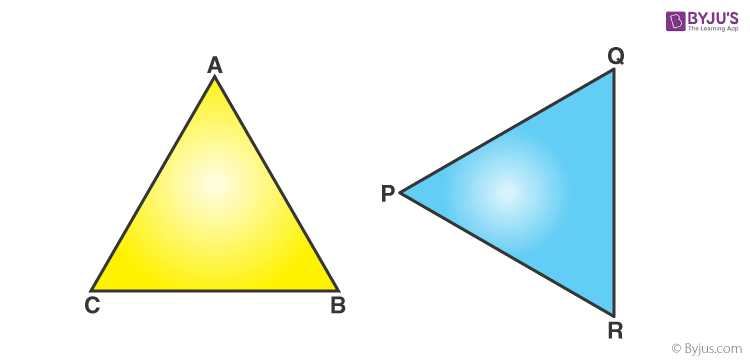# What do u mean by corresponding parts of congruent triangle (cpct)

A polygon made of three line segments forming three angles is known as a Triangle. Two triangles are said to be congruent if their sides have the same length and angles have the same measure. Thus, two triangles can be superimposed side to side and angle to angle.In the above figure, Δ ABC and Δ PQR are congruent triangles. This means,

Vertices:  A and P, B and Q, and C and R are the same.

Sides:  AB=PQ, QR= BC and AC=PR;

Angles:  ∠A = ∠P, ∠B = ∠Q, and ∠C = ∠R.

## What is CPCT?

CPCT stands for Corresponding parts of congruent triangles are congruent is a statement on congruent trigonometry. It states that if two or more triangles are congruent, then all of their corresponding angles and sides are as well congruent.

Corresponding Parts of Congruent Triangles (CPCT) are equal. While dealing with the concepts related to triangles and solving questions, we usually make use of the abbreviation cpct in short words rather than full form.

### CPCT Rules in Maths

Congruence can be predicted without actually measuring the sides and angles of a triangle. Different rules of congruency are as follows.

• SSS (Side-Side-Side)
• SAS (Side-Angle-Side)
• ASA (Angle-Side-Angle)
• AAS (Angle-Angle-Side)
• RHS (Right angle-Hypotenuse-Side)

#### For More Information On Introduction To Congruent Triangles, Watch The Below Video:### Articles to Explore:(151)(45)